# Graphics in qwraps2

There are several graphics generated within qwraps2. The naming convention for the “quick” plots was inspired by the ggplot2 function qplot. The development, flexibility, and robustness of these functions vary. Some “tips and tricks” are provided.

library(qwraps2)
packageVersion("qwraps2")
##  '0.5.2'
library(ggplot2)

# 1 qacf: Autocorrelation Plots

Generate an example data set.

set.seed(42)
n <- 250
x1 <- x2 <- x3 <- x4 <- vector('numeric', length = n)
x1 <- runif(1)
x2 <- runif(1)
x3 <- runif(1)
x4 <- runif(1)

# white noise
Z.1 <- rnorm(n, 0, 1)
Z.2 <- rnorm(n, 0, 2)
Z.3 <- rnorm(n, 0, 5)

for(i in 2:n)
{
x1[i] <- x1[i-1] + Z.1[i] - Z.1[i-1] + x4[i-1] - x2[i-1]
x2[i] <- x2[i-1] - 2 * Z.2[i] + Z.2[i-1] - x4[i-1]
x3[i] <- x3[i-1] + x2[i-1] + 0.2 * Z.3[i] + Z.3[i-1]
x4[i] <- x4[i-1] + runif(1, 0.5, 1.5) * x4[i-1]
}
testdf <- data.frame(x1, x2, x3, x4)

# Base acf plot for one variable
acf(testdf$x1)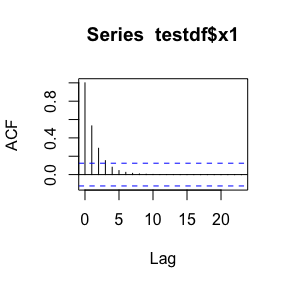# qacf plot for one variable qacf(testdf$x1)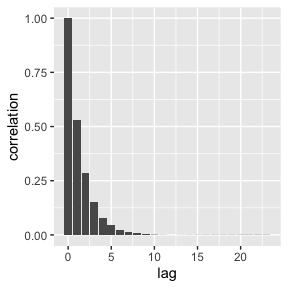qacf(testdf$x1, show_sig = TRUE)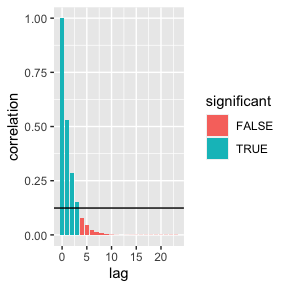# more than one variable acf(testdf)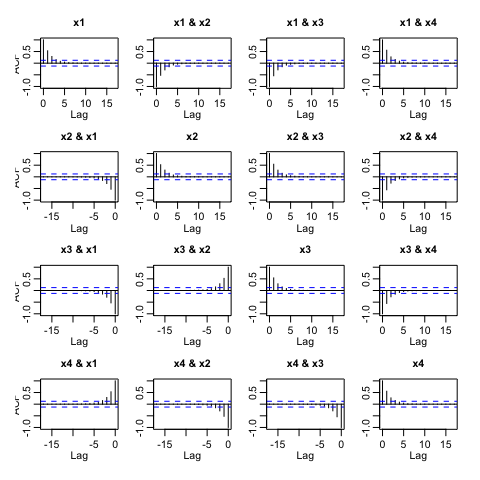qacf(testdf)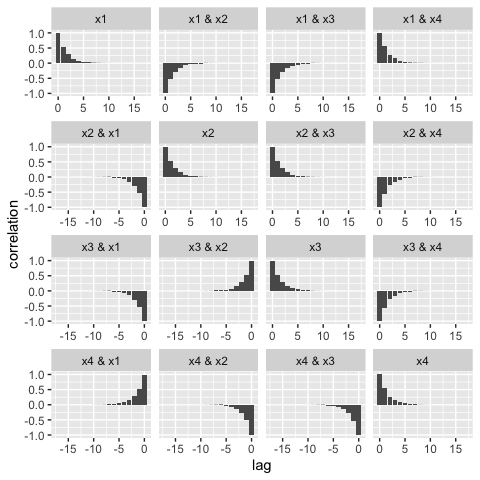qacf(testdf, show_sig = TRUE)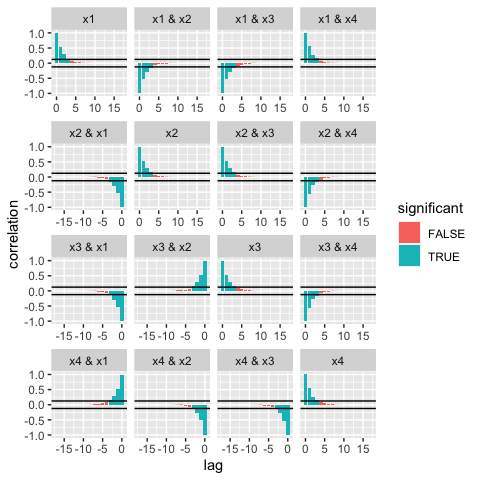## 1.1 Tips and tricks The implementation of qacf is based on the use of stats::acf to produce the statistics needed for the plot. If you want to get at the data itself to build your own acf plot you can extract the data frame from the qacf return: acf_plot_data <- qacf(testdf)$data
##   lag variable      value significant facets
## 1   0       V1 1.00000000        TRUE     x1
## 2   1       V1 0.53067306        TRUE     x1
## 3   2       V1 0.28669463        TRUE     x1
## 4   3       V1 0.15161298        TRUE     x1
## 5   4       V1 0.08015682       FALSE     x1
## 6   5       V1 0.04355315       FALSE     x1

# 2 qblandaltman: Bland Altman Plot

Introduced in [@altman1983measurement] and [@bland1986statistical], the qblandaltman method builds ggplot2 style Bland Altman plots. For examples we use the provided pefr data set which was transcribed from [@bland1986statistical]. See vignette("qwraps2-data-sets", package = "qwraps2") For more details on that data set.

The following replicates the figures in [@bland1986statistical].

Using the first measurement only:

pefr_m1 <-
cbind("Large" = pefr[pefr$measurement == 1 & pefr$meter == "Wright peak flow meter", "pefr"],
"Mini"  = pefr[pefr$measurement == 1 & pefr$meter == "Mini Wright peak flow meter", "pefr"])

A standard x-y style plot and a correlation coefficient suggests that the two meters provide reasonably similar results.

cor(pefr_m1)
##           Large      Mini
## Large 1.0000000 0.9432794
## Mini  0.9432794 1.0000000

qplot(x = pefr_m1[, 1],
y = pefr_m1[, 2],
geom = "point",
xlab = "Large Meter",
ylab = "Mini Meter",
xlim = c(0, 800),
ylim = c(0, 800)) +
geom_abline(slope = 1)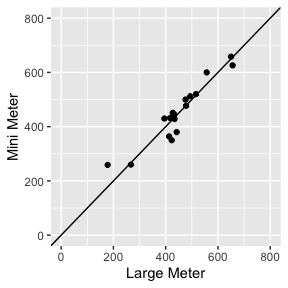However, for many reasons, this the above is misleading. One simple note: correlation is not a metric for agreement, i.e., perfect agreement would be shown if all the data points fell on the line of equality were as perfect correlation occurs when the data points are co-linear.

The Bland Altman plot plots the average value on the x-axis and the difference in the measurements on the y-axis:

qblandaltman(pefr_m1) +
xlim(0, 800) +
ylim(-100, 100) +
xlab("Average of two meters") +
ylab("Difference in the measurements")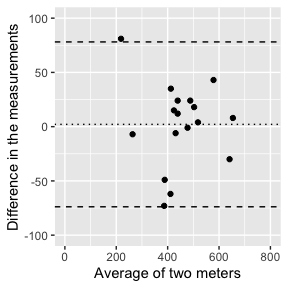There is no distinct relationship between the differences and the average, but the difference in the measurements between the two meters was observed to range between -73 and 81 liters per minute. Such a discrepancy between the meters is not observable from the simple x-y plot.

Reliability, or repeatability, of measurements can also be investigated with a Bland Altman plot.

pefr_mini <-
cbind(m1 = pefr[pefr$measurement == 1 & pefr$meter == "Mini Wright peak flow meter", "pefr"],
m2 = pefr[pefr$measurement == 2 & pefr$meter == "Mini Wright peak flow meter", "pefr"])

qblandaltman(pefr_mini)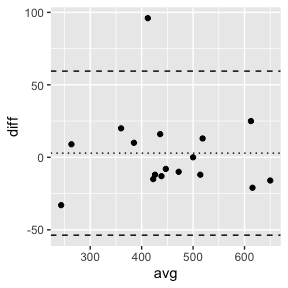# 3 qkmplot: Kaplan Meier Plots

# create a survfit object
require(survival)
leukemia.surv <- survival::survfit(survival::Surv(time, status) ~ x, data = survival::aml)

# base R km plot
survival:::plot.survfit(leukemia.surv, conf.int = TRUE, lty = 2:3, col = 1:2)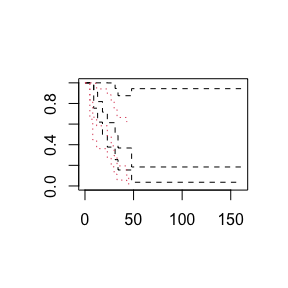# qkmplot
qkmplot(leukemia.surv, conf_int = TRUE)
## Warning: Removed 1 rows containing non-finite values (stat_step_ribbon).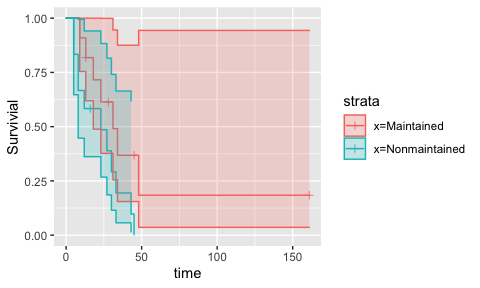# build a data.frame for plotting km curves, this could be helpful for
# creating bespoke plots
leukemia_km_data <- qkmplot_bulid_data_frame(leukemia.surv)
##   time n.risk n.event n.censor      surv upper     lower       strata
## 1    9     11       1        0 0.9090909     1 0.7541338 x=Maintained
## 2   13     10       1        1 0.8181818     1 0.6192490 x=Maintained
## 3   18      8       1        0 0.7159091     1 0.4884263 x=Maintained
qkmplot(leukemia_km_data)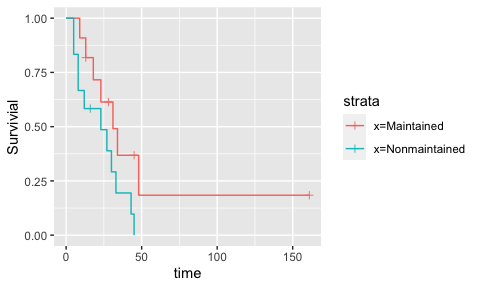# intercept only plot
intonly_fit <- survival::survfit(survival::Surv(time, status) ~ 1, data = survival::aml)
survival:::plot.survfit(intonly_fit, conf.int = TRUE)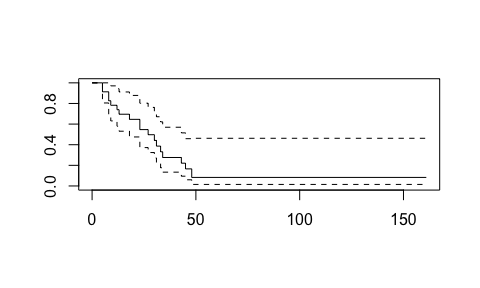qkmplot(intonly_fit, conf_int = TRUE)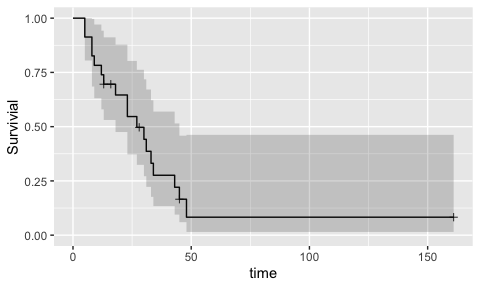# 4 qroc: Receiver Operating Curve

data(diamonds, package = "ggplot2")

# Create two logistic regression models
fit1 <- glm(I(price > 2800) ~ cut * color, data = diamonds, family = binomial())
fit2 <- glm(I(price > 2800) ~ cut + color + clarity, data = diamonds, family = binomial())

# Easiest way to get an ROC plot:
qroc(fit1)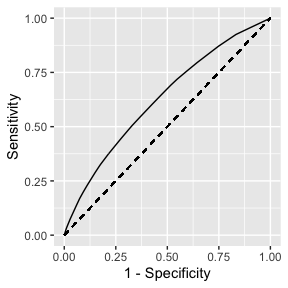qroc(fit2)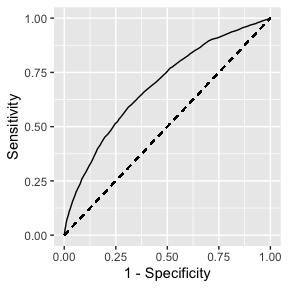# Create two data sets, this will also let you get the AUC out
data1 <- qroc_build_data_frame(fit1)
data2 <- qroc_build_data_frame(fit2)

auc(data1)
##  0.6268164
auc(data2)
##  0.6891337

# Plotting the ROC from the data set can be done too
qroc(data1)# Add the AUC value to the plot title
qroc(data2) + ggtitle(paste("Fit 2\nAUC =", round(auc(data2), 2)))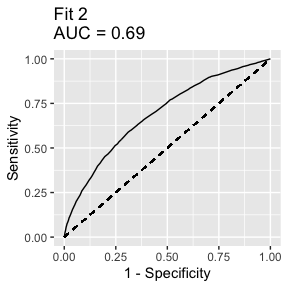# build a data set for plotting to ROCs on one plot
plot_data <- rbind(cbind(Model = "fit1", data1),
cbind(Model = "fit2", data2))
qroc(plot_data) + aes(color = Model)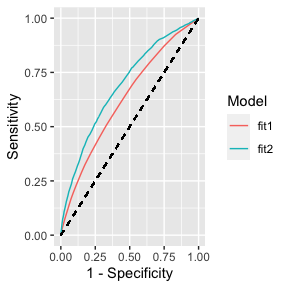# with AUC in the legend
plot_data <- rbind(cbind(Model = paste("Fit1\nauc =", round(auc(data1), 3)), data1),
cbind(Model = paste("Fit2\nauc =", round(auc(data2), 3)), data2))
qroc(plot_data) +
theme_bw() +
aes(color = Model, linetype = Model) +
theme(legend.position   = "bottom",
legend.text.align = 0.5)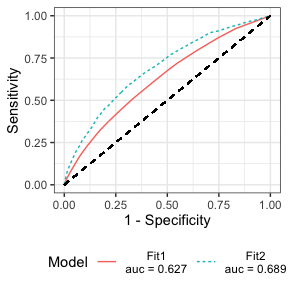# 5 Session Info

sessionInfo()
## R version 4.0.4 (2021-02-15)
## Platform: x86_64-apple-darwin17.0 (64-bit)
## Running under: macOS Big Sur 10.16
##
## Matrix products: default
## BLAS:   /Library/Frameworks/R.framework/Versions/4.0/Resources/lib/libRblas.dylib
## LAPACK: /Library/Frameworks/R.framework/Versions/4.0/Resources/lib/libRlapack.dylib
##
## locale:
##  C/en_US.UTF-8/en_US.UTF-8/C/en_US.UTF-8/en_US.UTF-8
##
## attached base packages:
##  stats     graphics  grDevices utils     datasets  methods   base
##
## other attached packages:
##  survival_3.2-7 ggplot2_3.3.3  qwraps2_0.5.2
##
## loaded via a namespace (and not attached):
##   Rcpp_1.0.6        highr_0.8         bslib_0.2.4       compiler_4.0.4
##   pillar_1.5.1      jquerylib_0.1.3   tools_4.0.4       digest_0.6.27
##   lattice_0.20-41   jsonlite_1.7.2    evaluate_0.14     lifecycle_1.0.0
##  tibble_3.1.0      gtable_0.3.0      debugme_1.1.0     pkgconfig_2.0.3
##  rlang_0.4.10      Matrix_1.3-2      DBI_1.1.1         yaml_2.2.1
##  xfun_0.21         withr_2.4.1       stringr_1.4.0     dplyr_1.0.5
##  knitr_1.31        generics_0.1.0    sass_0.3.1        vctrs_0.3.6
##  tidyselect_1.1.0  grid_4.0.4        glue_1.4.2        R6_2.5.0
##  fansi_0.4.2       rmarkdown_2.7     farver_2.1.0      purrr_0.3.4
##  magrittr_2.0.1    splines_4.0.4     scales_1.1.1      htmltools_0.5.1.1
##  ellipsis_0.3.1    assertthat_0.2.1  colorspace_2.0-0  labeling_0.4.2
##  utf8_1.1.4        stringi_1.5.3     munsell_0.5.0     crayon_1.4.1.9000